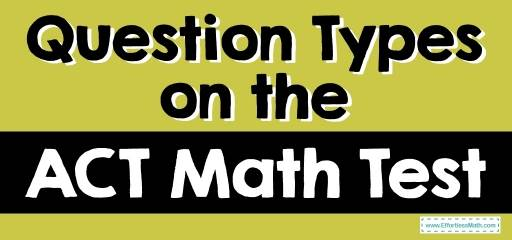# Question Types on the ACT Math TestACT testing is used to help colleges and universities get a standardized view of students’ knowledge in math, English, reading, science, and in some cases, writing. In this article, you will review what question types you will face on the ACT math test.

## What should you expect on the ACT Math Test?

The ACT math test consists of 60 multi-choice questions that you will have 60 minutes to complete. The questions assess the typical skills learned through grade 11.

The assumption is that you know basic formulas and computational skills, but you will not need to remember complex formulas or perform complex computations

The ACT math test is split into 8 areas of study.

The Absolute Best Book to Ace the ACT Math Test

## Preparing for Higher Mathematics (57% – 60%)

This section covers your more recent math learning, starting from your introduction to algebra as a way to deal with equations. It’s broken into 5 sub-sections:

### Number and Quantity (7% – 10%)

You will need knowledge of real and complex numbers. You will also need to understand numerical quantities in various ways, expressions with integer and rational exponents, vectors, and matrices.

### Algebra (12% – 15%)

You will need to solve, graph, and model multiple types of expressions. You will also need to understand different kinds of equations, linear, polynomial, radical, and exponential relationships. Finally, you will need to solve systems of equations and use them in real-world examples.

### Functions (12% – 15%)

You will need to use various functions, linear, radical, piecewise, polynomial, and logarithmic. You will also need to show you can manipulate and interpret functions. Finally, you will need to show you can use the features of graphs.

### Geometry (12% – 15%)

You will need to show you understand shapes and solids using concepts such as congruence and similarity relationships, surface area, and volume measurements. You will also need to use composite objects and solve missing values in various shapes. Finally, you will need to use trigonometric ratios and equations of conic sections.

### Statistics and Probability (8% – 12%)

You will need to understand the center and spread of distributions. You will need to use data collection methods. You will also need to use model relationships in bivariate data. Finally, you will need to calculate probabilities by using the related sample spaces.

## Integrating Essential Skills (40% – 43%)

This section checks your understanding of more complex problems. For example, you will need to understand and use rates and percentages, proportional relationships, area, surface area and volume, average and median, and express numbers differently.

There is also a sub-section on modeling, which checks your complete understanding of models.

Best ACT Math Prep Resource

## Math Formulas

The ACT math test doesn’t list any formulas to help answer the questions. Therefore, you may need an understanding of around 65 formulas to assist in getting a perfect score.

## ACT Calculator Policy

The ACT allows you to bring almost any 4-function, scientific, or graphing calculator. However, they provide a specific list of prohibited calculators. You don’t need a calculator to complete the test, but you should use any advantage you can get. You should also be familiar with the calculator you plan to use, don’t use one you’ve never used before.

## Final Thoughts

The ACT math test is not something you should be cramming. Instead, it’s testing your general understanding of all the knowledge you’ve been gaining in your math classes.

It would be beneficial if you brushed up on all areas listed above and ensure that you’ve memorized any formulas you require. In addition, You should understand what equipment, including which calculator, you can bring to the test.

Good luck!

The Best Books to Ace the ACT Math Test

## More from Effortless Math for ACT Test …

### Are you looking for a FREE ACT Math course to help you prepare for your test?

Check out our Ultimate ACT Math Course.

Have a look at our comprehensive ACT Math Worksheets to help you practice and prepare for the ACT Math test.

### Want to review the most common ACT Math formula?

Here is our complete list of ACT Math formulas.

### Looking for FREE ACT Math websites to find free online resources?

Here is our complete list of the Top 10 Free Websites for ACT Math Preparation.

Have a look at our Full-Length ACT Math Practice Test and Free ACT Math Practice Test.

### Do you know the key differences between SAT Math and ACT Math?

Find your answer here: SAT Math vs. ACT Math: the key differences.

The Perfect Prep Books for the ACT Math Test

## Have any questions about the ACT Test?

### What people say about "Question Types on the ACT Math Test - Effortless Math: We Help Students Learn to LOVE Mathematics"?

No one replied yet.

X
52% OFF

Limited time only!

Save Over 52%

SAVE \$40

It was \$76.99 now it is \$36.99